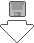### Requirements

• Psion/Pocket Book Palmtop

### What Is It?

XCalc is an enhancement for the 3/3a/3c calculator. It is divided into three sections:

1. Conversions
• Convert between imperial and metric, and a few more obsure conversions too
2. Mathematical Equations
• A very small section as yet, for those advanced mathematicians. If you're not studying maths though, you don't need to bother learning the commands
3. Physics Equations
• Similar to Mathematical Equations, but to work out equatons use commonly in Physicsxcalc.zipFull XCalc package